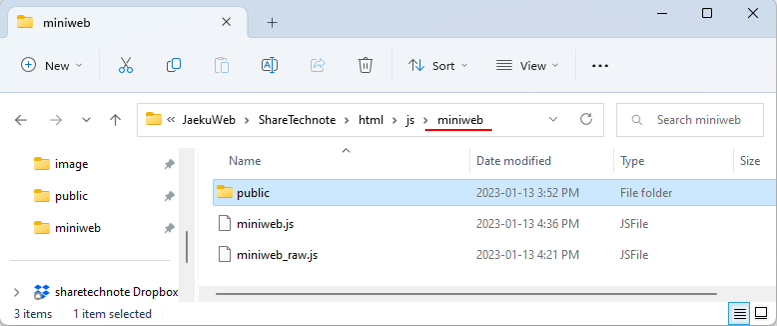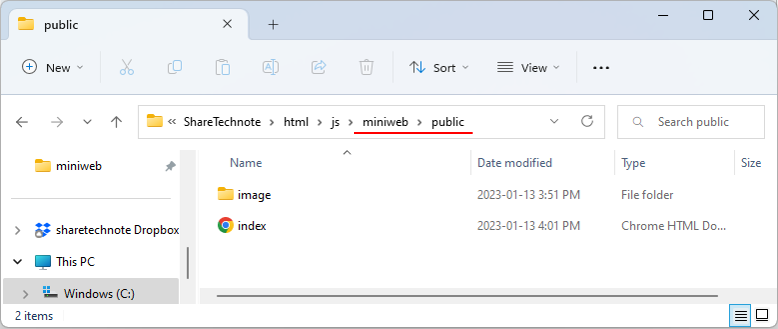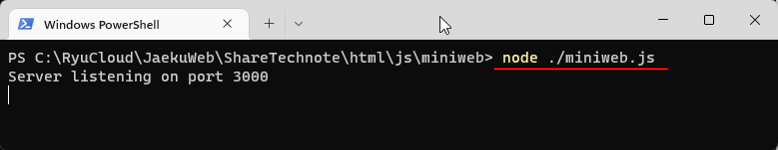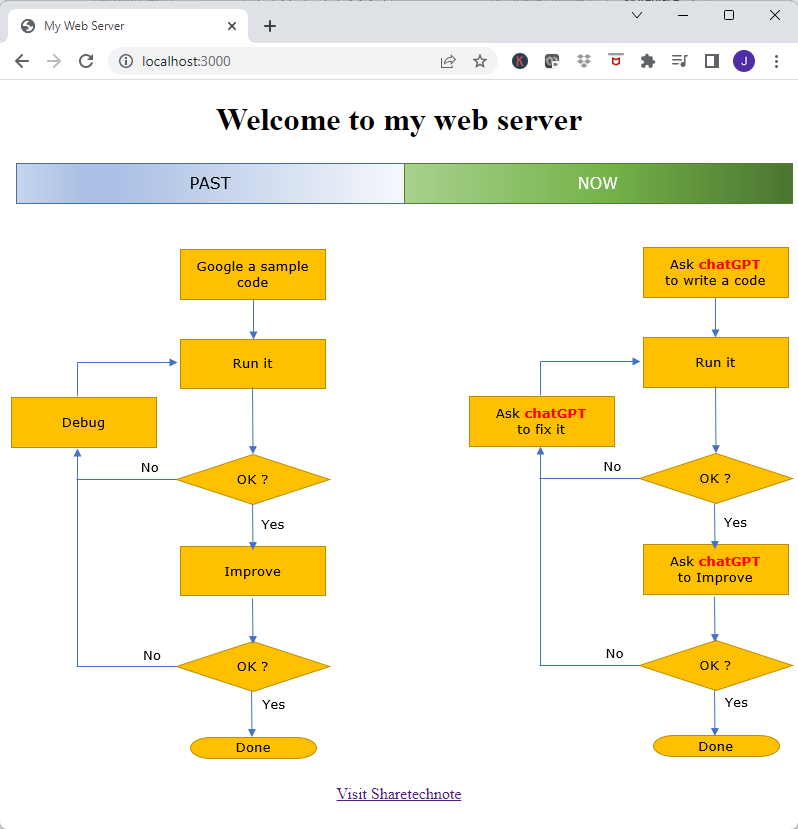Javascript

Web Server

This code is created first by chatGPT on Jan 13 2023 (meaning using chatGPT 3.5).

 can you write a simple web server script in java script without using express module. index.html should be located in public directory

Following is the final version of the code that I got after all the chats with chatGPT.

 miniweb_raw.js const http = require('http'); const fs = require('fs'); const path = require('path');   const PORT = 3000; const publicDir = 'public';   const handleRequest = (req, res) => {     // Build file path     const filePath = path.join(__dirname, publicDir, 'index.html');       // Check if file exists     fs.exists(filePath, (exists) => {         if (!exists) {             res.statusCode = 404;             res.end(`File \${filePath} not found!`);             return;         }           // Read file         fs.readFile(filePath, (err, data) => {             if (err) {                 res.statusCode = 500;                 res.end(`Error getting the file: \${err}.`);             } else {                 // If the file is found, set Content-type and send data                 res.setHeader('Content-type', 'text/html');                 res.end(data);             }         });     }); };   const server = http.createServer(handleRequest);   server.listen(PORT, () => {     console.log(`Server listening on port \${PORT}`); });

 can you rewrite the code using express module ?

 miniweb.js const express = require('express'); const app = express(); const path = require('path');   app.use(express.static(path.join(__dirname, 'public')));   app.get('/', (req, res) => {   res.sendFile(path.join(__dirname, 'public', 'index.html')); });   app.listen(3000, () => {   console.log('Server running on port 3000'); });

 can you add a routine for printing out client information ?

 miniweb.js const express = require('express'); const path = require('path'); const requestIp = require('request-ip');   const app = express(); const PORT = 3000; const publicDir = 'public';   app.use(requestIp.mw());   app.get('/', (req, res) => {     console.log(`Client IP: \${req.clientIp} - Requested: \${req.url}`);     res.sendFile(path.join(__dirname, publicDir, 'index.html')); });   app.use(express.static(path.join(__dirname, publicDir)));   app.listen(PORT, () => {     console.log(`Server listening on port \${PORT}`); });